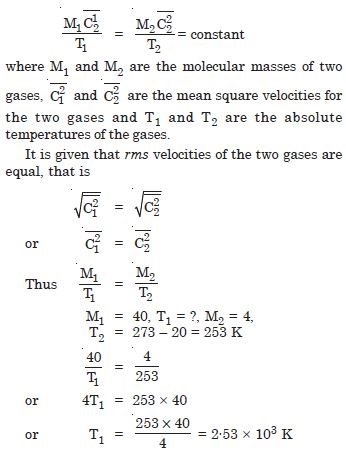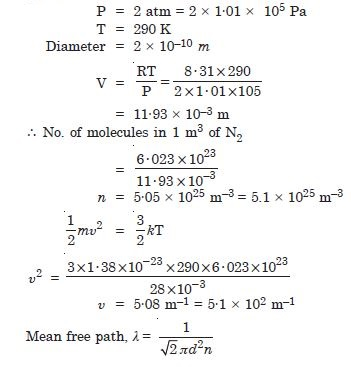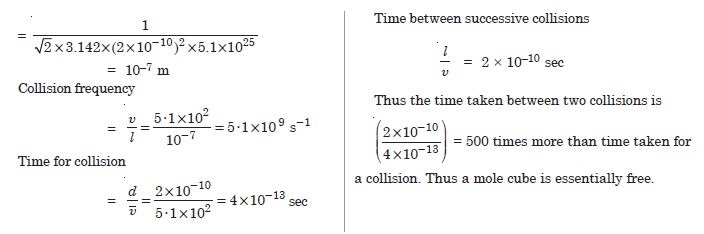# NCERT Solutions for Class 11 Physics Chapter 13 - Kinetic Theory

##### Question 1:

Estimate the fraction of molecular volume to the actual volume occupied by oxygen gas at STP. Take the size of oxygen molecule to be roughly 3Å.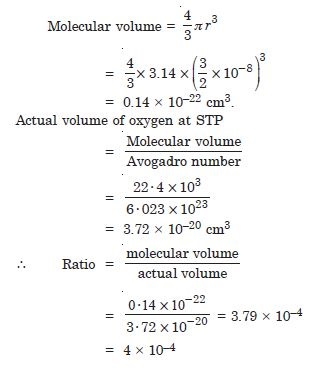##### Question 2:

Molar volume is the volume occupied by 1 mol of any (ideal) gas at standard temperature and pressure (S.T.P.) 1 atmospheric pressure, (0$°$C). Show that it is 22.4 litres.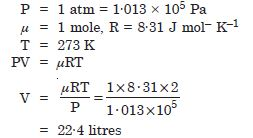##### Question 3:

Figure 13.2 shows plots of PV/T versus P for 1·00 × 10–3 kg of oxygen gas at two different temperatures.
What does the dotted plot signify ?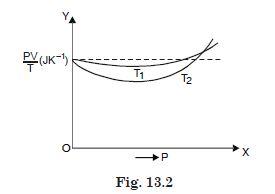The dotted plot corresponds to ideal gas behaviour,

##### Question 4:

Figure 13.2 shows plots of PV/T versus P for 1·00 × 10–3 kg of oxygen gas at two different temperatures.
Which is true :
T1 > T2 or T1 < T2 ?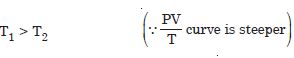##### Question 5:

Figure 13.2 shows plots of PV/T versus P for 1·00 × 10–3 kg of oxygen gas at two different temperatures.
What is the value of PV/T, where the curves meet on the y-axis ?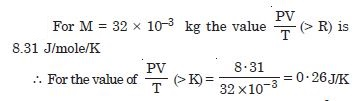##### Question 6:

Figure 13.2 shows plots of PV/T versus P for 1·00 × 10–3 kg of oxygen gas at two different temperatures.
If we obtain similar plots for 1·00 × 10–3 kg of hydrogen, would we get the same value of PV/T at the point where the curves meet on the y-axis ? If not, what mass of hydrogen yields the same value of PV/T (for low pressure-high temperature region of the plot) (Molecular mass of H2 = 2·02 of Oxygen = 32·0 u, R = 8·31 J mol–1K––1) ?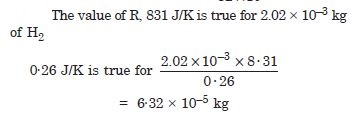##### Question 7:

An oxygen cylinder of volume 30 litres has initial gauze pressure of 15 atm and a temperature of 27$°$ C. After some oxygen is withdrawn from the cylinder the gauze pressure drop to 11 atm. and its temperature drops to 17$°$ C. Estimate the mass of oxygen taken out of the cylinder. (R = 8·3 J mol–1 K–1) Molecular mass of O2 = 32.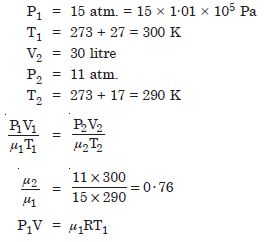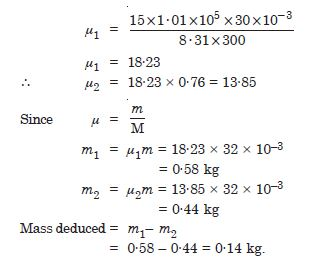##### Question 8:

An air bubble of volume of 1.0 cm3 rises from the bottom of lake 40 m deep at a temperature of 12$°$ C. To what volume does it grow when it reaches the surface which is at a temperature of 35$°$C ?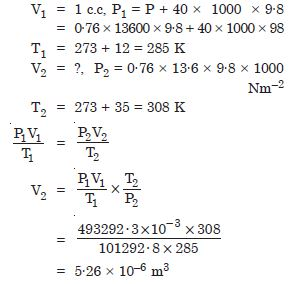##### Question 9:

Estimate the total number of air molecules (inclusive oxygen, nitrogen, water vapour and other constituents) in a room of capacity 25·0 m3 at a temperature of 27$°$ C and 1 atm. pressure. (KB = 1·38 × 10–23 JK–1)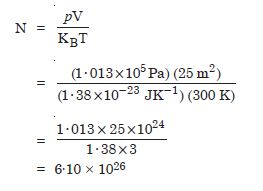##### Question 10:

Estimate the average thermal energy of a helium atom (i) room temperature (27$°$C), (ii) the temperature on the surface of the star (6000$°$ K), (iii) the temperature of 10 million degree kelvin (the typical core temperature in the case of a star). KB = 1.38 × 10–23 JK–1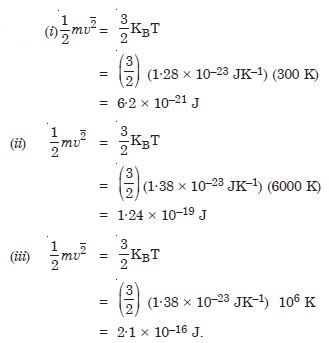##### Question 11:

Three vessels of equal capacity have gases at the same temperature and pressure. The first vessel contains neon (monoatomic), the second contains chlorine (diatomic) and the third contains uranium hexafluoride (polyatomic). Do the vessels contain equal number of respective molecules ? Is the root mean squared speed of molecules the same in the three cases ? If not in which case is $\mathrm{\upsilon }$rms the largest ?

According to Avogadro’s law “equal volumes of all gases contain equal number of molecules at the same temperature and pressure. Hence three vessels contain equal number of respective molecules.
No. The root mean square speed of molecules is not the same in the three cases.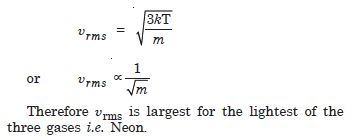##### Question 12:

At what temperature is the root mean square speed of an atom in an argon gas equal to the rms speed of a helium gas atom at – 20$°$C ? (Atomic mass of Ar = 39·9u, of He = 4·0)